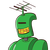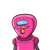# if the lcm of two number is 18 the hcf is 3 and one of the number is 6 than find the second number​

if the lcm of two number is 18 the hcf is 3 and one of the number is 6 than find the second number​

### 2 thoughts on “if the lcm of two number is 18 the hcf is 3 and one of the number is 6 than find the second number​”

1.9

Step-by-step explanation:

18 × 3 / 6

18 × 1/2

9

9 is the second number.

2.